Next: Active Memory Up: A Basis for Long-Range Previous: Simulation Results

# The ACTION Network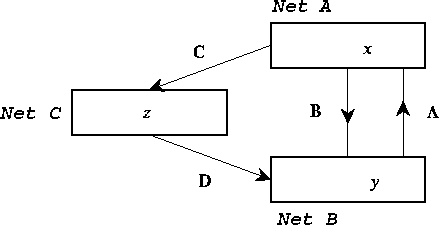Figure 17: Schematic of the ACTION net, showing the three modules A (cortex), B (thalamus) and C (basal ganglia) with activities x, y and z respectively. The connection matrices A, B, C and D are also shown.

The basic structure of the ACTION network is shown in Figure 17. It involves three interconnected modules. The first of these, denoted A in the Figure, is the frontal cortex. This is represented as a single module in the Figure, but is really representing any one of the set of loops known to preserve topology on circulation of activity round the frontal cortex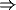basal gangliathalamusfrontal cortex . The second net, B, is the region of thalamus appropriate for the particular frontal loop considered. Finally there is the basal ganglia C, shown in the Figure as a single layer. However that is only a simplification for the more complex two-layered system of which it is actually composed. The purpose of the model of the figure is to extract the most crucial features of the more complex structure; the disinhibitory action of the basal ganglia on the thalamus will be preserved by the model. At the same time, there is assumed to be lateral inhibition on the basal ganglia layer C. Thus two of the most important features of the basal ganglia are incorporated in the model of Figure 17. The general connectivity being assumed between the modules of Figure 17 is topographic, with an exponential spread of a small amount. Thus the action of the modules A and B on each other will be excitatory, as is the feedforward action of A on C and C on B. The action of the neurons in C on B will not be assumed adaptive, due to their having arisen from inhibitory neurons in the globus pallidus.

It is assumed that the time taken for a nerve impulse to travel directly from A to B (and vice versa) is one unit, and from A to B by way of C to be 2n time units (so n time units for each of the passages from A to C and C to B). In actuality, these times are respectively about 4 msec and 30 msec , so that n would be at least 3. The connection matrices from B to A is denoted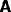, from A to B is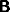, from A to C,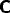and C to B denoted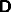. Lateral connection is assumed to occur on the basal ganglia, and will be denoted by a connection matrix. The activities of the modules A, B, C are denoted respectively by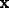,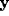, and. The simplest equations for the development of activity in the coupled modules are thus the update equationswhere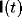and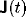are external inputs (from heteromodal cortex or the limbic system) arriving at the frontal cortex and basal ganglia respectively, and the sigmoidal functions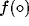,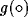, and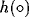are the output functions of the nodes A, B, and C respectively. These functions will be assumed to have the general form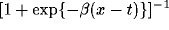for an activityon the node in question, where t is the threshold, and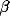an inverse temperature. The possibility of any of these functions being linear will be considered later. It will be necessary to include various extra features in order to make ACTION have full neurobiological realism. However the first and simplest model, that of Figure 17 and equation (27), will hopefully be sufficient to give a broad basis for modelling the features mentioned earlier --- namely, active memory, comparator action, sequence generation, and attentional control.

There is now the question of determining the nature of the response of the system (27) to the inputs I and J. There are a large range of nets specified by this structure, since there is a great choice of the connection matrices,,,,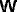and. The interconnectivity between the different modules has been assumed to be topographic, so that each of the corresponding connection matrices,,andwill be assumed to be nearly diagonal. It is clear that the lateral connection matrices are not of that form. They will be considered in more detail later, but will initially be neglected.

An important question, not contained explicitly in the ACTION network assumptions outlined above, is that of the coding of information by the networks concerned. The most accessible of these seems to be for cortex, where there is known topographic representations of the body in the motor cortex. In that case, it seems that locally there is a population vector coding , in which there is a random distribution of preferred vectors for movement of a local region. The scale of the modelling in ACTION is such that only the population vector is observable, so such a randomisation will not be necessary. In any case, the lateral spread of the connection matrices should not cause problems of continued looping of activity by such randomisations.Next: Active Memory Up: A Basis for Long-Range Previous: Simulation Results